# Transportation Problem | Set 2 (NorthWest Corner Method)

• Difficulty Level : Hard
• Last Updated : 26 May, 2021

An introduction to Transportation problem has been discussed in the previous article, in this article, finding the initial basic feasible solution using the NorthWest Corner Cell Method will be discussed.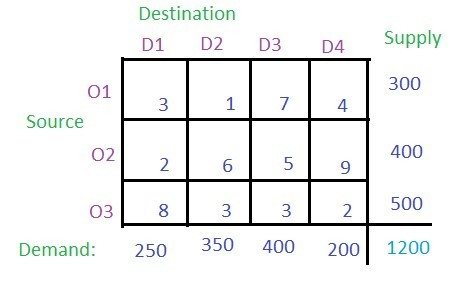Explanation: Given three sources O1, O2 and O3 and four destinations D1, D2, D3 and D4. For the sources O1, O2 and O3, the supply is 300, 400 and 500 respectively. The destinations D1, D2, D3 and D4 have demands 250, 350, 400 and 200 respectively.

Solution: According to North West Corner method, (O1, D1) has to be the starting point i.e. the north-west corner of the table. Each and every value in the cell is considered as the cost per transportation. Compare the demand for column D1 and supply from the source O1 and allocate the minimum of two to the cell (O1, D1) as shown in the figure.
The demand for Column D1 is completed so the entire column D1 will be canceled. The supply from the source O1 remains 300 – 250 = 50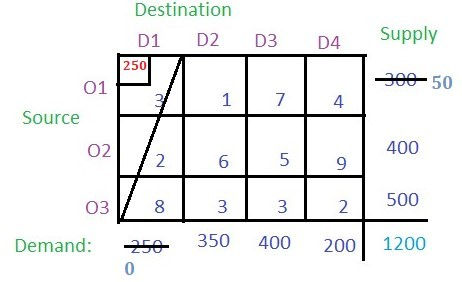Now from the remaining table i.e. excluding column D1, check the north-west corner i.e. (O1, D2) and allocate the minimum among the supply for the respective column and the rows. The supply from O1 is 50 which is less than the demand for D2 (i.e. 350), so allocate 50 to the cell (O1, D2). Since the supply from row O1 is completed cancel the row O1. The demand for column D2 remain 350 – 50 = 300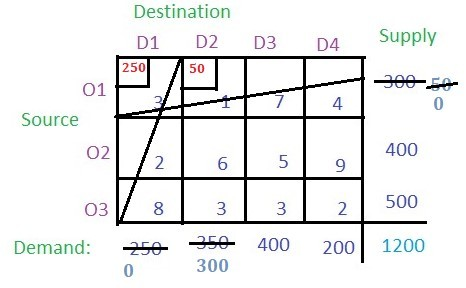From the remaining table the north-west corner cell is (O2, D2). The minimum among the supply from source O2 (i.e 400) and demand for column D2 (i.e 300) is 300, so allocate 300 to the cell (O2, D2). The demand for the column D2 is completed so cancel the column and the remaining supply from source O2 is 400 – 300 = 100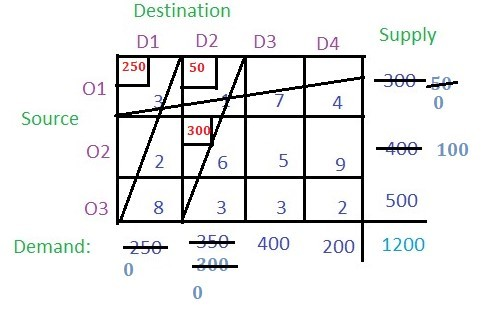Now from remaining table find the north-west corner i.e. (O2, D3) and compare the O2 supply (i.e. 100) and the demand for D2 (i.e. 400) and allocate the smaller (i.e. 100) to the cell (O2, D2). The supply from O2 is completed so cancel the row O2. The remaining demand for column D3 remains 400 – 100 = 300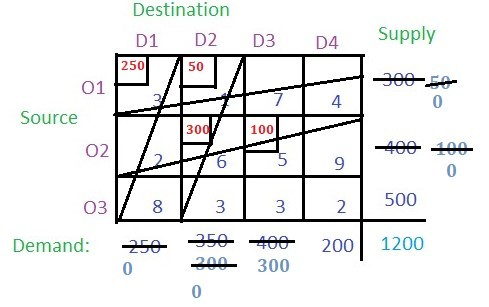Proceeding in the same way, the final values of the cells will be: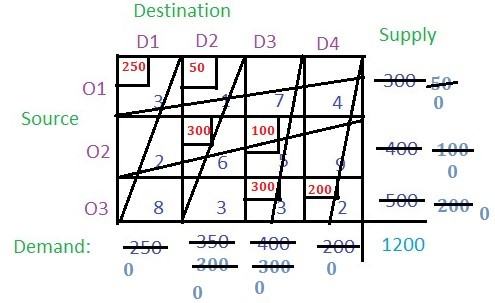Note: In the last remaining cell the demand for the respective columns and rows are equal which was cell (O3, D4). In this case, the supply from O3 and the demand for D4 was 200 which was allocated to this cell. At last, nothing remained for any row or column.
Now just multiply the allocated value with the respective cell value (i.e. the cost) and add all of them to get the basic solution i.e. (250 * 3) + (50 * 1) + (300 * 6) + (100 * 5) + (300 * 3) + (200 * 2) = 4400

My Personal Notes arrow_drop_up